# Chemical Equation MCQ - 1 (Advanced)

## 10 Questions MCQ Test Chemistry for JEE Advanced | Chemical Equation MCQ - 1 (Advanced)

Description
Attempt Chemical Equation MCQ - 1 (Advanced) | 10 questions in 30 minutes | Mock test for JEE preparation | Free important questions MCQ to study Chemistry for JEE Advanced for JEE Exam | Download free PDF with solutions
QUESTION: 1

### X,Y and Z react in the 1 : 1 : 1 stoichiometric ratio. The concentration of X, Y  and Z where found to vary with time as shown in the figure below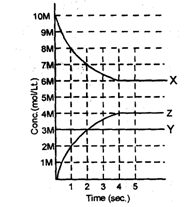Q. Which of the following equilibrium reaction represents the correct variation of concentration with time.

Solution:

Clearly concentration of Y is not changing with the time hence it will be pure solid or liquid. Concentration of X decreasing hence it will be in reactant and Z will be in product.

QUESTION: 2

### X,Y and Z react in the 1 : 1 : 1 stoichiometric ratio. The concentration of X, Y  and Z where found to vary with time as shown in the figure belowQ. Value of the equilibrium constant (Kc) for the equilibrium represented in above sketch will be.

Solution: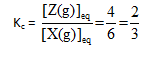QUESTION: 3

### X,Y and Z react in the 1 : 1 : 1 stoichiometric ratio. The concentration of X, Y  and Z where found to vary with time as shown in the figure belowQ. If above equilibrium is established in a 2L container by taking reactants in sufficient amount then how many moles of component Y must have reacted to establish the equilibrium.

Solution:

From the graph we can see that 4 mole/litre of Z is formed therefore      4 × 2 moles of solid will be required.

QUESTION: 4

For certain substances such as ammonium chloride, nitrogen peroxide, phosphorus               pentachloride, etc. the measured densities are found to be less than those calculated from their molecular formula. The observed densities decrease towards a limit as the temperature is raised. This is due to the splitting of the molecules into simpler ones. The process is reversible and is called thermal dissociation.

Examples :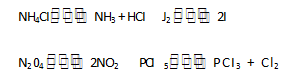With increase in the number of molecules, the volume increases (pressure  remaining constant) and, in consequence, the density decreases. As the temperature rises, more and more dissociation takes place, and when practically complete dissociation occursthe density reaches its lowest limit.The extent of issociation, ice., the fraction of the total number of molecules which suffers dissociation is called the degree of dissociation. Gas density measurements can be used to determine the degree of dissociation. Let us take by general case where one molecule of a substance A splits up into n molecule of B on heating; i.e.,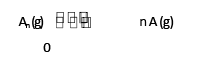t = 0 a

t = teq       a  — x               nx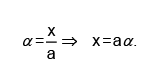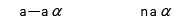Total no. of moles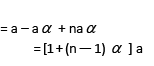Observed molecular weight or molar mass of the mixture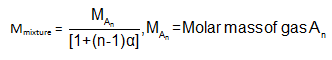Q.

A sample of mixture of A(g), B(g) and C(g) under equilibrium has a mean molecular weight (observed) is 80.

The equilibrium is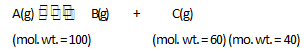Find the degree of dissociation α for A(g).

Solution: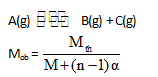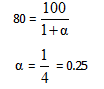QUESTION: 5

For certain substances such as ammonium chloride, nitrogen peroxide, phosphorus               pentachloride, etc. the measured densities are found to be less than those calculated from their molecular formula. The observed densities decrease towards a limit as the temperature is raised. This is due to the splitting of the molecules into simpler ones. The process is reversible and is called thermal dissociation.

Examples :With increase in the number of molecules, the volume increases (pressure  remaining constant) and, in consequence, the density decreases. As the temperature rises, more and more dissociation takes place, and when practically complete dissociation occursthe density reaches its lowest limit.The extent of issociation, ice., the fraction of the total number of molecules which suffers dissociation is called the degree of dissociation. Gas density measurements can be used to determine the degree of dissociation. Let us take by general case where one molecule of a substance A splits up into n molecule of B on heating; i.e.,t = 0 a

t = teq       a  — x               nxTotal no. of molesObserved molecular weight or molar mass of the mixtureQ.

If the total mass of the mixture in the above case is 300 gm, the moles at C(g) present are.

Solution:

Mols of C = a α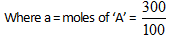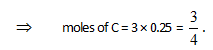QUESTION: 6

For certain substances such as ammonium chloride, nitrogen peroxide, phosphorus               pentachloride, etc. the measured densities are found to be less than those calculated from their molecular formula. The observed densities decrease towards a limit as the temperature is raised. This is due to the splitting of the molecules into simpler ones. The process is reversible and is called thermal dissociation.

Examples :With increase in the number of molecules, the volume increases (pressure  remaining constant) and, in consequence, the density decreases. As the temperature rises, more and more dissociation takes place, and when practically complete dissociation occursthe density reaches its lowest limit.The extent of issociation, ice., the fraction of the total number of molecules which suffers dissociation is called the degree of dissociation. Gas density measurements can be used to determine the degree of dissociation. Let us take by general case where one molecule of a substance A splits up into n molecule of B on heating; i.e.,t = 0 a

t = teq       a  — x               nxTotal no. of molesObserved molecular weight or molar mass of the mixtureQ.

The K for the reaction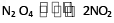is 640 mm at 775 K. The percentage dissociation of N2O4 at equilibrium pressure of 160 mm is :

Solution: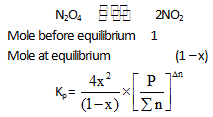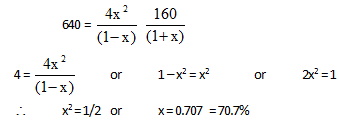QUESTION: 7

For certain substances such as ammonium chloride, nitrogen peroxide, phosphorus               pentachloride, etc. the measured densities are found to be less than those calculated from their molecular formula. The observed densities decrease towards a limit as the temperature is raised. This is due to the splitting of the molecules into simpler ones. The process is reversible and is called thermal dissociation.

Examples :With increase in the number of molecules, the volume increases (pressure  remaining constant) and, in consequence, the density decreases. As the temperature rises, more and more dissociation takes place, and when practically complete dissociation occursthe density reaches its lowest limit.The extent of issociation, ice., the fraction of the total number of molecules which suffers dissociation is called the degree of dissociation. Gas density measurements can be used to determine the degree of dissociation. Let us take by general case where one molecule of a substance A splits up into n molecule of B on heating; i.e.,t = 0 a

t = teq       a  — x               nxTotal no. of molesObserved molecular weight or molar mass of the mixtureQ.

x (degree of dissociation) varies with D/d in the above reaction according to :

Solution: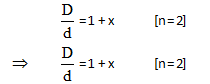QUESTION: 8

For certain substances such as ammonium chloride, nitrogen peroxide, phosphorus               pentachloride, etc. the measured densities are found to be less than those calculated from their molecular formula. The observed densities decrease towards a limit as the temperature is raised. This is due to the splitting of the molecules into simpler ones. The process is reversible and is called thermal dissociation.

Examples :With increase in the number of molecules, the volume increases (pressure  remaining constant) and, in consequence, the density decreases. As the temperature rises, more and more dissociation takes place, and when practically complete dissociation occursthe density reaches its lowest limit.The extent of issociation, ice., the fraction of the total number of molecules which suffers dissociation is called the degree of dissociation. Gas density measurements can be used to determine the degree of dissociation. Let us take by general case where one molecule of a substance A splits up into n molecule of B on heating; i.e.,t = 0 a

t = teq       a  — x               nxTotal no. of molesObserved molecular weight or molar mass of the mixtureQ.

The equation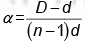is correctly matched for :

Solution: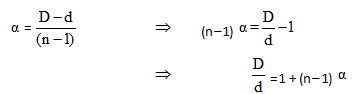Hence one mole of reactant should produce total n moles of product.

QUESTION: 9

Match the following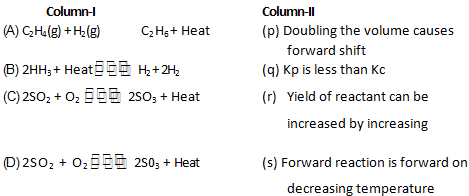Solution:
QUESTION: 10

Match the following (multiple)

Left column : Represents an equilibrium situratton through a chemical equation and below each equation a stimulus is given which may or may not

disturb the equilibrium situration.

Right coloumn : Represents the responses immediately after the    disturbance is created.

With                                                                      R1 : Rate of forward reaction.

Rb Rate of backward reaction.

Q : Reaction quotient

K : Equilibrium constant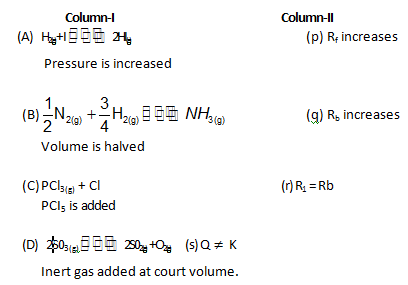Solution:Use Code STAYHOME200 and get INR 200 additional OFF Use Coupon Code